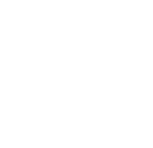NCERT Solutions Class 10 Maths – Triangles | Chapter 6 Exercise – 6.5 Question 3

Triangles | Chapter 6 Exercise 6.5 Question 3:

In the following figure, ABD is a triangle right angled at A and AC ⊥ BD. Show that

(i) AB2 = BC × BD       (ii) AC2 = BC × DC      (iii) AD2 = BD × CDSolution: Detailed explanation of exercise 6.5 question 3 is given in the below image and YouTube video.